## ISRO CS 2015

 Question 1
Which of the given number has its IEEE-754 32 bit floating point representation as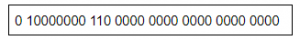A 2.5 B 3 C 3.5 D 4.5
Digital-Logic-Design       Number-Systems
Question 1 Explanation:
→ Sign bit S = 0 (It means positive number)
→ E=1000 0000B = 128D (in normalized form)
→ Fraction is 1.11B (with an implicit leading 1) = 1 + 1×2-1 + 1×2-2
= 1.75D
→ The number is +1.75 × 2(128-127)
= +3.5D
 Question 2
The range of integers that can be represented by an n bit 2’s complement number system is:
 A – 2n – 1 to 2n – 1 – 1 B – (2n – 1 – 1) to (2n – 1 – 1) C – 2n – 1 to 2n – 1 D – (2n – 1 + 1) to (2n – 1 – 1)
Digital-Logic-Design       Number-Systems
Question 2 Explanation:
In 2’s complement numbers, the range of integers are from -2n-1 to 2n-1 – 1
 Question 3
How many 32K X 1 RAM chips are needed to provide a memory capacity of 256K-bytes?
 A 8 B 32 C 64 D 128
Digital-Logic-Design       RAM
Question 3 Explanation:
Number of RAM chips required = Memory capacity / RAM chip capacity.
=(256K * 8) / (32K x 1)
=(256*1024*8) / (32*1024*1)
=64
 Question 4
A modulus -12 ring counter requires a minimum of
 A 10 flip-flops B 12 flip-flops C 8 flip-flops D 6 flip-flops
Digital-Logic-Design       Sequential-Circuits
Question 4 Explanation:
A ring counter is a type of counter composed of flip-flops connected into a shift register, with the output of the last flip-flop fed to the input of the first, making a "circular" or "ring" structure.
The “MODULO” or “MODULUS” of a counter is the number of states the counter counts or sequences through before repeating itself and a ring counter can be made to output any modulo number. A “mod-n” ring counter will require “n” number of flip-flops connected together to circulate a single data bit providing “n” different output states
So, modulus-12 requires , 12 flip-flops.
 Question 5
The complement of the Boolean expression AB ( B’C + AC ) is
 A ( A’ + B’ ) + ( B + C’ )( A’ + C’ ) B ( A’ + B’ ) + ( BC’ + A’C’ ) C ( A’ + B’ )( B + C’) + ( A + C’ ) D ( A + B )( B’ + C )( A + C )
Digital-Logic-Design       Boolean-Expression
Question 5 Explanation:
→ Finding complement boolean expression, we require Demorgan’s law.
→ DeMorgan’s law and after complementing:
⇒ ( AB ( B’C + AC ))’
⇒ (A + B)’ + (B’C + AC)’
⇒ (A’ + B’) + ( B’C)’ (AC)’
⇒ (A’ + B’) + ( B + C’)(A’ + C’)
 Question 6
The code which uses 7 bits to represent a character is
 A ASCII B BCD C EBCDIC D Gray
Digital-Logic-Design       Number-Systems
Question 6 Explanation:
→ ISO/IEC 646, like ASCII, is a 7-bit character set. It does not make any additional codes available, so the same code points encoded different characters in different countries. Escape codes were defined to indicate which national variant applied to a piece of text, but they were rarely used, so it was often impossible to know what variant to work with and, therefore, which character a code represented, and in general, text-processing systems could cope with only one variant anyway.
→ Extended Binary Coded Decimal Interchange Code (EBCDIC) is an 8-bit binary code for numeric and alphanumeric characters.
→ BCD encoding uses 4 bits to represent each digit from the range 0 to 9 in its binary form.
→ In case of Gray codes, any number of bits can be used to represent a character, according to the requirement.
 Question 7
If half adders and full adders are implemented using gates, then for the addition of two 17 bit numbers (using minimum gates) the number of half adders and full adders required will be
 A 0, 17 B 16, 1 C 1, 16 D 8, 8
Question 7 Explanation:
1. An adder is a digital circuit that performs addition of numbers. The half adder adds two binary digits called as augend and addend and produces two outputs as sum and carry; XOR is applied to both inputs to produce sum and AND gate is applied to both inputs to produce carry.
2. The full adder adds 3 one bit numbers, where two can be referred to as operands and one can be referred to as bit carried in. And produces 2-bit output, and these can be referred to as output carry and sum.
Half adder is used to add two numbers of the least significant bits, so one half adder is required. In order to add remaining 16 bits of two numbers , we require 16 full adders
 Question 8
Minimum number of 2x1 multiplexers required to realize the following function,
f = A’B’C + A’B’C’
Assume that inputs are available only in true form and Boolean constant 1 and 0 are available.
 A 1 B 2 C 3 D 7
Digital-Logic-Design       Combinational-Circuit
Question 8 Explanation:
Given function f = A’B’C + A’B’C’
=A’B’(C+C’) (C+C’=1)
=A’B’
=(A+B)’
So we can implement with minimum Two number of 2x1 multiplexers
 Question 9
The number of 1’s in the binary representation of (3*4096 + 15*256 + 5*16 + 3) are:
 A 8 B 9 C 10 D 12
Digital-Logic-Design       Number-Systems
Question 9 Explanation:
Binary expression of (3*4096 + 15*256 + 5*16 + 3)
=(12,288+3840+80+3)
=(16211)10
=‭(0011111101010011‬)2
Total number of 1’s in binary representation is 10.
 Question 10
The boolean expression AB + AB’+ A’C + AC is independent of the boolean variable
 A A B B C C D None of these
Digital-Logic-Design       Boolean-Expression
Question 10 Explanation:→ AB + AB' + A'C + AC
= A(B + B') + C(A + A')
= A+C
As the expression is independent of 'B'
 Question 11
If the sequence of operations – push (1), push (2), pop, push (1), push (2), pop, pop, pop, push (2), pop are performed on a stack, the sequence of popped out values
 A 2,2,1,1,2 B 2,2,1,2,2 C 2,1,2,2,1 D 2,1,2,2,2
Data-Structures       Queues-and-Stacks
Question 11 Explanation:Final Pop sequence: 22112
 Question 12
A machine needs a minimum of 100 sec to sort 1000 names by quick sort. The minimum time needed to sort 100 names will be approximately
 A 50.2 sec B 6.7 sec C 72.7 sec D 11.2 sec
Algorithms       Sorting
Question 12 Explanation:
We need to find the minimum time to sort the names by using quick sort.
The Worst case time complexity is O(n2)
Average and best case time complexity is O(n logn)
Step-1: Time taken to sort 1000 names by quicksort
100= c*nlogn
= c*1000log1000
= 100
c=1/10log1000
Now, time taken to sort 100 names by quicksort,
=c*nlogn
= (1/10log1000)*100*log100
= 6.7
 Question 13
Six files F1, F2, F3, F4, F5 and F6 have 100, 200, 50, 80, 120, 150 records respectively. In what order should they be stored so as to optimize act. Assume each file is accessed with the same frequency
 A F3, F4, F1, F5, F6, F2 B F2, F6, F5, F1, F4, F3 C F1, F2, F3, F4, F5, F6 D Ordering is immaterial as all files are accessed with the same frequency.
Algorithms       Greedy-approach
Question 13 Explanation:
Optimal merge pattern will give the optimal result after performing sorted order. Every time it will take least frequency elements and performing merging.
→ Final order is F3, F4, F1, F5, F6, F2
 Question 14
A hash table with ten buckets with one slot per bucket is shown in the following figure. The symbols S1 to S7 initially entered using a hashing function with linear probing.The maximum number of comparisons needed in searching an item that is not present isA 4 B 5 C 6 D 3
Data-Structures       Hashing
Question 14 Explanation:
In this, maximum size of cluster = 4 (S6, S3, S7, S1)
→ Worst case of finding a number is equal to maximum size of cluster + 1(after searching all the cluster it enters into empty cluster)
→ Maximum no. of comparisons = 4+1
= 5
 Question 15
The queue data structure is to be realized by using stack. The number of stacks needed would be
 A It cannot be implemented B 2 stacks C 4 stacks D 1 stack
Data-Structures       Queues-and-Stacks
Question 15 Explanation:
Step-1: Pop elements from Stack-1 and push into Stack-2.
For this,
int x=element=stack-1,pop();
stack-2.push(element);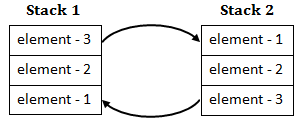Step-2: Once the complete stack-1 gets copied to Stack-2, then we can simply call pop() on s2, it will remove the element-1.
So, A queue can be implemented using 2 stacks.
 Question 16
Consider the following Relationship Entity Diagram(ERD)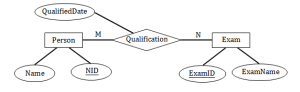Which of the following possible relations will not hold if the above ERD is mapped into a relation model?
 A Person (NID, Name) B Qualification (NID, ExamID, QualifiedDate) C Exam (ExamID, NID, ExamName) D Exam (ExamID, ExamName)
Database-Management-System       ER-Model
Question 16 Explanation:
Given diagram is ER diagram , So any object, for example, entities, attributes of an entity, relationship sets, and attributes of relationship sets, can be represented with the help of an ER diagram.
We need to convert ER diagram to relational model.
There are two entities in the ER diagram named Person and Exam with attributes which are represented in ellipse.
Table name: PersonNow Create table for a relationship
Add the primary keys of all participating Entities as fields of table with their respective data types.
If relationship has any attribute, add each attribute as field of table.
Declare a primary key composing all the primary keys of participating entities.
Declare all foreign key constraints.
Table for “Qualification” relationshipQuestion 17
Consider the following log sequence of two transactions on a bank account, with initial balance 12000, that transfer 2000 to a mortgage payment and then apply a 5% interest.
1. T1 start

2. T1 B old=1200 new=10000

3. T1 M old=0 new=2000

4. T1 commit

5. T2 start

6. T2 B old=10000 new=10500

7. T2 commit

Suppose the database system crashes just before log record 7 is written. When the system is restarted, which one statement is true of the recovery procedure?
 A We must redo log record 6 to set B to 10500 B We must undo log record 6 to set B to 10000 and then redo log records 2 and 3 C We need not redo log records 2 and 3 because transaction T1 has committed D We can apply redo and undo operations in arbitrary order because they are idempotent.
Database-Management-System       Transactions
Question 17 Explanation:
→ When the database system crashes after the transactions have committed then we need to redo the log records.
→ And if the database system crashes before the transactions have committed then we need to undo the log records.
So from above theory we can say that option (B) is the correct Solution.
 Question 18
Given a block can hold either 3 records or 10 key pointers. A database contains n records, then how many blocks do we need to hold the data file and the dense index
 A 13n/30 B n/3 C n/10 D n/30
Database-Management-System       Indexing
Question 18 Explanation:
Total number of records in the data base=n
Given block will hold either 3 records or 10 key pointers.
Number of blocks to store “n” records = n/3
Number of blocks to store dense file index = n/10
Total blocks to hold data file and dense index = n/3 + n/10 = 13n/30 blocks.
 Question 19
The correct syntax to write “Hi there” in Javascript is
 A jscript.write (“Hi There”); B document.write (“Hi There”); C print (“Hi There”); D print.jscript (“Hi There”);
Web-Technologies       Java-Script
Question 19 Explanation:
JavaScript can "display" data in different ways:
Writing into an HTML element, using innerHTML.
Writing into the HTML output using document.write().
Writing into an alert box, using window.alert().
Writing into the browser console, using console.log().
 Question 20
Let R = (A, B, C, D, E, F) be a relation schema with the following dependencies C→ F, E→ A, EC→ D, A→ B. Which of the following is a key of R?
 A CD B EC C AE D AC
Database-Management-System       Functional-Dependency
Question 20 Explanation:
Here, simple way to solve this question is,
First we have to find the right hand side values of a dependencies. Check whether it cover all variables in a relation .
In right hand side, CE are missing. It means definitely CE should be there in candidate key.
(CE)+=(A,B,C,D,E,F).
Remaining all are not satisfying candidate key properties.
 Question 21
Consider the following code fragment
void foo(int x, int y)
{
x+=y;
y+=x;
}
main()
{
int x=5.5;
foo(x,x);
}
What is the final value of x in both call by value and call by reference, respectively?
 A 5 and 16 B 5 and 12 C 5 and 20 D 12 and 20
Programming-for-Output-Problems       Pointers
Question 21 Explanation:
Call by Value:
The call by value method of passing arguments to a function copies the actual value of an argument into the formal parameter of the function. In this case, changes made to the parameter inside the function have no effect on the argument.
foo(x,x) ---->foo(5,5)
Modification of x and y values won’t effect in the main program, So still x will hold value “5” because the data type is integer.
Call by Reference:
The call by reference method of passing arguments to a function copies the address of an argument into the formal parameter. Inside the function, the address is used to access the actual argument used in the call. It means the changes made to the parameter affect the passed argument.
Function call foo(x,x)---foo(5,5) calls the function
void foo(int x, int y)
{
x+=y;
y+=x;
}
Here x and y variables, both pointing to same variable “x” in the main function.
So, if any modification done in the function it automatically reflect in the main function.
x+=y means x=x+y=5+5=10 // The variable “x” of main function will hold value 10 which used by both local variables of x and y
y+=x means u=y+x= 10+10=20 // Y value is 10 because , it point to variable ”x” in the main function.
 Question 22
Consider the following relational schema:
Suppliers( sid:integer, sname:string, city:string, street:string )
Parts( pid:integer, pname:string, color:string)
Catalog( sid:integer, pid:integer, cost:real)
Consider the following relational query on the above database:
SELECT S.sname
FROM Suppliers S
WHERE S.sid NOT IN (SELECT C.sid FROM Catalog C
WHERE C.pid NOT IN (SELECT P.pid FROM Parts P
WHERE P.color<> 'blue'))
Assume that relations corresponding to the above schema are not empty. Which one of the following is the correct interpretation of the above query?
 A Find the names of all suppliers who have supplied a non-blue part. B Find the names of all suppliers who have not supplied a non-blue part C Find the names of all suppliers who have supplied only non blue parts. D Find the names of all suppliers who have not supplied only non-blue parts.
Database-Management-System       SQL
Question 22 Explanation: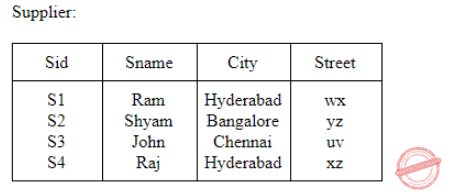If we execute the given query the output will be S3 and S4 i.e., names of all suppliers who didn’t supply blue parts which is option (A).
Option (D) says names of suppliers who didn’t supply only blue parts that means, supplier should supply all other parts for sure and shouldn’t supply blue part.
 Question 23
Consider the following schema:
Emp (Empcode, Name, Sex, Salary, Deptt)
A simple SQL query is executed as follows:
SELECT Deptt FROM Emp WHERE sex = 'M' GROUP by Dept Having avg (Salary) > {select avg (Salary) from Emp}
The output will be
 A Average salary of male employee is the average salary of the organization B Average salary of male employee is less than the average salary of the organization C Average salary of male employee is equal to the average salary of the organization D Average salary of male employee is more than the average salary of the organization
Database-Management-System       SQL
Question 23 Explanation:
Query-1: select avg (Salary) from Emp:
This query will give average salary of all the all employes.
Query-2:SELECT Deptt FROM Emp WHERE sex = 'M' GROUP by Dept Having avg (Salary)
Average salary of employee who is male where we grouping by department
Query-2 > Query-1
Average salary of male employee is more than the average salary of the organization
 Question 24
Given the following expression grammar:
E→ E*F | F+E |F
F→ F-F | id
Which of the following is true?
 A * has higher precedence than + B – has higher precedence than * C + and – have same precedence D + has higher precedence than *
Compiler-Design       Associativity-and-Precedence
Question 24 Explanation:
The operator which is in low level that can have high preference.
Order of precedence is *, +, -.
Here * and + have equal preference, '-' can have higher precedence than + and *.
 Question 25
The number of tokens in the following C statement is
printf(“i=%d, &i=%x”, i&i);
 A 13 B 6 C 10 D 0
Compiler-Design       Compilers
Question 25 Explanation:Question 26
Which grammar rules violate the requirement of the operator grammar? A, B, C are variables and a, b, c are terminals 1) A → BC 2) A → CcBb 3) A → BaC 4) A → ε
 A 1 only B 1 and 2 only C 1 and 3 only D 1 and 4 only
Compiler-Design       Parsers
Question 26 Explanation:
Operator values doesn't contains nullable values and two adjacent non-terminals on RHS production.
i) On RHS it contains two adjacent non-terminals.
ii) Have nullable values.
 Question 27
Which one of the following is a top-down parser?
 A Recursive descent parser B Shift left associative parser C SLR(k) parser D LR(k) parser
Compiler-Design       Parsers
Question 27 Explanation: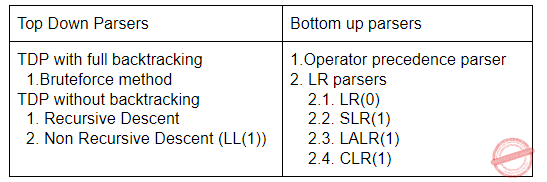Question 28
YACC stands for
 A yet accept compiler constructs B yet accept compiler compiler C yet another compiler construct D yet another compiler compiler
Compiler-Design       Parsers
Question 28 Explanation:
→ Yacc (Yet Another Compiler-Compiler) is a computer program for the Unix operating system developed by Stephen C. Johnson.
→ It is a Look Ahead Left-to-Right (LALR) parser generator, generating a parser, the part of a compiler that tries to make syntactic sense of the source code, specifically a LALR parser, based on an analytic grammar written in a notation similar to Backus-Naur Form (BNF)
 Question 29
Which statement is true?
 A LALR parser is more powerful and costly as compare to other parsers B All CFG’s are LP and not all grammars are uniquely defined C Every SLR grammar is unambiguous but not every unambiguous grammar is SLR D LR(K) is the most general backtracking shift reduce parsing method
Compiler-Design       Parsers
Question 29 Explanation:

Option-A: LR > LALR > SLR

Canonical LR parser is more powerful than LALR parser. So, it FALSE

Option-B: Here, LP is linear precedence. Every grammar generated by LP are CFG but all CFG's are not LP. So, it is false

Option-C: TRUE

Option-D: LR(K) is general non backtracking shift reduce parsing method. So, It is false
 Question 30
Semaphores are used to solve the problem of
I. Race Condition
II. Process Synchronization
III. Mutual Exclusion
IV. None of the above
 A I and II B II and III C All of the above D None of the above
Operating-Systems       Process-Synchronization
Question 30 Explanation:
Semaphore are used to solve the problem of process synchronization and mutual exclusion.
Semaphore are 2 types.
Counting semaphore
Binary semaphore
 Question 31
If there are 32 segments, each size 1K bytes, then the logical address should have
 A 13 bits B 14 bits C 15 bits D 16 bits
Operating-Systems       Memory-Management
Question 31 Explanation:
Given data, total 32 segments
Each segment size is 1K bytes.
Find the logical address=?
32 segments=25
Segment size=210
⇒ 25*210
⇒ 215
So, 15 bits are required.
 Question 32
In a lottery scheduler with 40 tickets, how we will distribute the tickets among 4 processes and such that each process gets 10%, 5%, 60% and 25% respectively?A a B b C c D d
Operating-Systems       Process-Scheduling
Question 32 Explanation:
Total number of tickets =40
Total 4 processors: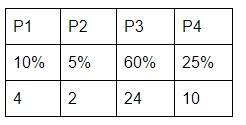Question 33
Suppose a system contains n processes and system uses the round-robin algorithm for CPU scheduling then which data structure is best suited for ready queue of the process
 A stack B queue C circular queue D tree
Operating-Systems       CPU-Scheduling
Question 33 Explanation:
→ This type of scheduling is one of the very basic algorithms for Operating Systems in computers which can be implemented through circular queue data structure.
→ Round-robin (RR) is one of the algorithms employed by process and network schedulers in computing.
→ As the term is generally used, time slices (also known as time quanta) are assigned to each process in equal portions and in circular order, handling all processes without priority (also known as cyclic executive).
→ Round-robin scheduling is simple, easy to implement, and starvation-free.
→ Round-robin scheduling can also be applied to other scheduling problems, such as data packet scheduling in computer networks.
 Question 34
A hard disk system has the following parameters : Number of tracks = 500 Number of sectors/track = 100 Number of bytes /sector = 500 Time taken by the head to move from one track to adjacent track = 1 ms Rotation speed = 600 rpm. What is the average time taken for transferring 250 bytes from the disk ?
 A 300.5 ms B 255.5 ms C 255 ms D 300 ms
Computer-Organization       Secondary-Memory
Question 34 Explanation:
Avg. time to transfer = Avg. seek time + Avg. rotational delay + Data transfer time
For Avg. seek time:
Given that, time to move between successive tracks is 1ms.
Time to move from track1 to track1 = 0ms
Time to move from track1 to track2 = 1ms
Time to move from track1 to track3 = 2ms
⋮ Time to move from track1 to track500 = 499ms
∴ Avg. seek time = 0+1+2+...+499/500 = 249.5ms
Avg. rotational delay:
600 rotations in 60sec.
One rotation takes 60/600 s = 100ms ∴ Avg. rotational delay = 100/2 = 50ms

Data transfer time:
In one rotation, we can read data on one complete track
= 100 × 500 = 50,000B data is read in one complete rotation
One complete rotation takes 100ms.
100ms → 50,000B
250B → 100/50000 × 250 = 0.5ms
∴ Avg. time to transfer = 249.5 × 50 + 0.5 = 300ms
 Question 35
At a particular time of computation the value of a counting semaphore is 7. Then 20 P operations and 15 V operations were completed on this semaphore. The resulting value of the semaphore is :
 A 42 B 2 C 7 D 12
Operating-Systems       Process-Synchronization
Question 35 Explanation:
Counting semaphore value is 7
After 20 P operations value of semaphore = 7 – 20 = -13
After 15 V operations value of semaphore = -13 + 15 = 2
 Question 36
Increasing the RAM of a computer typically improves performance because:
 A Virtual Memory increases B Larger RAMs are faster C Fewer page faults occur D Fewer segmentation faults occur
Operating-Systems       Memory-Management
Question 36 Explanation:
→ When page frames increases, then no. of page faults decreases.
→ Such as if RAM size increases, then no. of page entries increases, then no. of page faults decreases.
 Question 37
Consider the following program.
main()
{
fork();
fork();
fork();
}
How many new processes will be created?
 A 8 B 6 C 7 D 5
Operating-Systems       System-Calls
Question 37 Explanation:
The no. of child process created = 2n -1
= 23 -1
= 7
(where n is number of fork() statements)
 Question 38
Consider the following C declaration
struct
{
short s;
union
{
float y;
long z;
}u;
}t;
Assume that the objects of the type short, float and long occupy 2 bytes, 4 bytes and 8 bytes, respectively. The memory requirement for variable t, ignoring alignment consideration, is
 A 22 bytes B 18 bytes C 14 bytes D 10 bytes
Programming-for-Output-Problems       Structure
Question 38 Explanation:
short  = 5×2 = 10 max[float, long] = max [4, 8] = 8 Total = short + max[float,long] = 10+8 = 18
 Question 39
If a node has K children in B-tree, then the node contains exactly _____ keys.
 A K2 B K – 1 C K + 1 D K1/2
Database-Management-System       B-and-B+-Trees
Question 39 Explanation:
According to Knuth's definition, a B-tree of order m is a tree which satisfies the following properties: Every node has at most m children. Every non-leaf node (except root) has at least ceil(m/2) children. The root has at least two children if it is not a leaf node. A non-leaf node with k children contains k−1 keys. All leaves appear in the same level, and carry information.
 Question 40
The time complexity of the following C function is (assume n > 0)
int recursive (int n)
{
if(n == 1)
return (1);
else
return (recursive (n-1) + recursive (n-1));
}
 A O(n) B O(n log n) C O(n2) D O(2n)
Algorithms       Time-Complexity
Question 40 Explanation:
T(n) = 2T (n - 1) + 1
= 2 [2T(n - 2) + 1] + 1
= 22 T(n - 2) + 3

= 2kT(n - k) + (2k - 1)
= 2n-1T(1) + (2n-1 - 1)
= 2n-1 + 2n-1 - 1
= 2n - 1
≅ O( 2n )
 Question 41
The number of spanning tree for a complete graph with 7 vertices are:
 A 25 B 75 C 35 D 22×5
Algorithms       Greedy-approach
Question 41 Explanation:
To find number of spanning trees of a complete graph is nn-2.
Here, there are 7 nodes.
=77-2
=75
=16,807
 Question 42
If one uses straight two-way merge sort algorithm to sort the following elements in ascending order:
20, 47, 15, 8, 9, 4, 40, 30, 12, 17
then the order of these elements after second pass of the algorithm is:
 A 8, 9, 15, 20, 47, 4, 12, 17, 30, 40 B 8, 15, 20, 47, 4, 9, 30, 40, 12, 17 C 15, 20, 47, 4, 8, 9, 12, 30, 40, 17 D 4, 8, 9, 15, 20, 47, 12, 17, 30, 40
Algorithms       Sorting
Question 42 Explanation:
Explanation:Question 43
Let R1 and R2 be regular sets defined over the alphabet, then
 A R1 ∩ R2 is not regular B R1 ∪ R2 is not regular C Σ * – R1 is regular D R1 * is not regular
Theory-of-Computation       Regular-Language
Question 43 Explanation:
Regular languages are closed under,
1) Intersection
2) Union
3) Complement
4) Kleene-closure
Σ* - R1 is the complement of R1.
 Question 44
The DNS maps the IP address to
 A A binary address as strings B An alphanumeric address C A hierarchy of domain names D A hexadecimal address
Question 44 Explanation:
→ DNS maps the IP addresses into a hierarchy of domain names.
→ DNS is a host name to IP address translation service.
→ DNS is a distributed database implemented in a hierarchy of name servers.
→ It is an application layer protocol for message exchange between clients and servers.
 Question 45
To add a background color for all h1 elements, which of the following HTML syntax is used
 A h1 { background-color :#FFFFFF} B { background-color :#FFFFFF} . h1 C h1 { background-color :#FFFFFF} . h1(all) D h1. all{bgcolor= #FFFFFF}
Web-Technologies       HTML
Question 45 Explanation:
→ Select and style all

# elements AND all elements: Example: h1, p { background-color: yellow; } → To style several elements with the same style, separate each element name with a comma.

 Question 46
To declare the version of XML, the correct syntax is
 A B <*xml version='1.0'/> C D
Web-Technologies       HTML
Question 46 Explanation:
1.XML stands for eXtensible Markup Language.
2.XML was designed to store and transport data.
3.XML was designed to be both human- and machine-readable.
Syntax:
 Question 47
A T-switch is used to
 A Control how messages are passed between computers B Echo every character that is received C Transmit characters one at a time D Rearrange the connections between computing equipments
Question 47 Explanation:
A T-switch is used to rearrange the connections between computing equipments
 Question 48
What frequency range is used for microwave communications, satellite and radar?
 A Low frequency: 30 kHz to 300 kHz B Medium frequency: 300 kHz to 3 MHz C Medium frequency: 300 kHz to 3 MHz D Extremely high frequency: 30000 kHz
Question 48 Explanation:
→ Microwave signals are often divided into three categories:
1)Ultra high frequency (UHF) (0.3-3 GHz);
2)Super high frequency (SHF) (3-30 GHz); and
3)Extremely high frequency (EHF) (30-300 GHz).
→ In addition, microwave frequency bands are designated by specific letters.
→ The term “P band” is sometimes used for ultra high frequencies below the L-band. For other definitions, see Letter Designations of Microwave Bands
→ Lower Microwave frequencies are used for longer links, and regions with higher rain fade. Conversely, Higher frequencies are used for shorter links and regions with lower rain fade.
 Question 49
How many bits internet address is assigned to each host on a TCP/IP internet which is used in all communication with the host?
 A 16 bits B 32 bits C 48 bits D 64 bits
Computer-Networks       TCP/IP
Question 49 Explanation:
→ Present we are using IPv4 address, it has 32 bits to communicate with the host.
→ Planning to shift IPv6 address, it has 128 bits to communicate with the host
 Question 50
How many characters per sec (7 bits + 1 parity) can be transmitted over a 2400 bps line if the transfer is synchronous ( 1 start and 1 stop bit)?
 A 300 B 240 C 250 D 275
Computer-Organization       Synchronous-and-asynchronous-Communication
Question 50 Explanation:
Given bandwidth is 2400 bps
Each character consists of (7+1)bits = 8 bits
Synchronous transmission is a data transfer method which is characterized by a continuous stream of data in the form of signals which are accompanied by regular timing signals which are generated by some external clocking mechanism meant to ensure that both the sender and receiver are synchronized with each other.
Once sender and receiver synchronized means no need to use start and stop bits every time
Total number of characters can be transmitted per second is =bandwidth/ number of bits in each character=2400/8 = 300
 Question 51
In CRC if the data unit is 100111001 and the divisor is 1011 then what is dividend at the receiver?
 A 100111001101 B 100111001011 C 100111001 D 100111001110
Computer-Networks       CRC
Question 51 Explanation:
→ A cyclic redundancy check (CRC) is an error-detecting code commonly used in digital networks and storage devices to detect accidental changes to raw data. Blocks of data entering these systems get a short check value attached, based on the remainder of a polynomial division of their contents. On retrieval, the calculation is repeated and, in the event the check values do not match, corrective action can be taken against data corruption. CRCs can be used for error correction
→ Given data unit is 100111001 and divisor is 1011
→ The polynomial is written in binary as the coefficients; a 3rd-degree polynomial has 4 coefficients (1x3 + 0x2 + 1x + 1). In this case, the coefficients are 1, 0, 1 and 1. The result of the calculation is 3 bits long.
→ First padded with zeros corresponding to the bit length n of the CRC. Here we used 3-bit CRC
→ After padding zeros to the data unit, Data unit = 100111001000
→ Divide data unit by divisor→ Append the remainder(011) to the data unit where zero’s are padded Then data unit becomes 100111001011 at receiver end
 Question 52
An ACK number of 1000 in TCP always means that
 A 999 bytes have been successfully received B 1000 bytes have been successfully received C 1001 bytes have been successfully received D None of the above
Computer-Networks       TCP/IP
Question 52 Explanation:
TCP sequence numbers and Acknowledgments: 1. A Sequence number is sent from the host and is total number of bytes sent UP to this point, during the conversation, not including the current payload
2. The acknowledgement number would be the total number of bytes received by the destination, FROM the sender, plus one (i.e. the one being the next byte it expects to get). Initial Sequence number is needed in order to identify how many number of bytes received by receiver.
So the answer is option-D because of not mentioning of sequence number.
 Question 53
In a class B subnet, we know the IP address of one host and the mask as given below:
 A 125.134.96.0 B 125.134.112.0 C 125.134.112.66 D 125.134.0.0
Question 53 Explanation:
Given
IP address in binary Form = 01111101 10000110 01110000 01000010
Subnet Mask in binary Form = 11111111 11111111 11100000 00000000
Every IP address has Network id and Host id,
We will get Network id by doing bitwise -AND (&) operation with IP address and Subnet MaskQuestion 54
A certain population of ALOHA users manages to generate 70 request/sec. If the time is slotted in units of 50 msec, then channel load would be
 A 4.25 B 3.5 C 350 D 450
Computer-Networks       Network-Access-Methods
Question 54 Explanation:
The number of requests generated by ALOHA users are 70 request/sec
The amount of time slotted in unit is 50 msec
The number of time slots for generating user requests in 1 second are 1000/50=20
The Channel load can be calculated dividing number of requests to the time slots Channel Load = 70/20=3.5
 Question 55
Which statement is false?
 A PING is a TCP/IP application that sends datagrams once every second in the hope of an echo response from the machine being PINGED B If the machine is connected and running a TCP/IP protocol stack, it should respond to the PING datagram with a datagram of its own C If PING encounters an error condition, an ICMP message is not returned D PING display the time of the return response in milliseconds or one of several error message
Computer-Networks       PING
Question 55 Explanation:
→ Ping operates by sending Internet Control Message Protocol (ICMP) echo request packets to the target host and waiting for an ICMP echo reply.
→ The program reports errors, packet loss, and a statistical summary of the results, typically including the minimum, maximum, the mean round-trip times, and standard deviation of the mean.
 Question 56
A router uses the following routing table:A packet bearing a destination address 144.16.68.117 arrives at the router. On which interface will it be forwarded?
 A eth0 B eth1 C eth2 D eth3
Question 56 Explanation: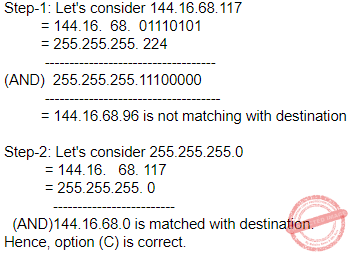Question 57
Which layers of the OSI reference model are host-to-host layers?
 A Transport, session, presentation, application B Session, presentation, application C Datalink, transport, presentation, application D Physical, datalink, network, transport
Computer-Networks       OSI-TCP-layers
Question 57 Explanation: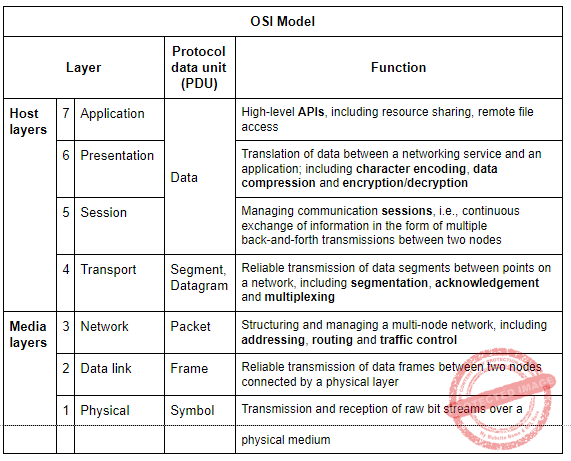Question 58
Alpha and Beta testing are forms of
 A Acceptance testing B Integration testing C System testing D Unit testing
Software-Engineering       Software-testing
Question 58 Explanation:
Acceptance testing can mean one of two things:
A smoke test is used as a build acceptance test prior to further testing, e.g., before integration or regression.
Acceptance testing performed by the customer, often in their lab environment on their own hardware, is known as user acceptance testing (UAT). Acceptance testing may be performed as part of the hand-off process between any two phases of development.
Alpha testing:
Alpha testing is simulated or actual operational testing by potential users/customers or an independent test team at the developers' site
. Alpha testing is often employed for off-the-shelf software as a form of internal acceptance testing before the software goes to beta testing.
Beta testing
Beta testing comes after alpha testing and can be considered a form of external user acceptance testing. Versions of the software, known as beta versions, are released to a limited audience outside of the programming team known as beta testers.
The software is released to groups of people so that further testing can ensure the product has few faults or bugs. Beta versions can be made available to the open public to increase the feedback field to a maximal number of future users and to deliver value earlier, for an extended or even indefinite period of time (perpetual beta).
 Question 59
The decimal number has 64 digits.The number of bits needed for its equivalent binary representation is?
 A 200 B 213 C 246 D 277
Digital-Logic-Design       Number-Systems
Question 59 Explanation:
Consider three digits(1,2,3) of decimal numbers.Maximum number, we can generate by that three digits are 103-1 which is 999.
Then, Decimal number has 64 digits, so maximum number is 1064-1
Similarly, in the binary representation with “n” bits the maximum number is 2n-1
So we can write 1064 –1 = 2n – 1 --->1064 = 2n
After taking log2 on both sides
log22n=log21064
n log22=64 log 210
n=64*(3.322) [ log22=1 & log210 =3.322]
n=212.608
n=213
 Question 60
In X = (M + N x O)/(P x Q), how many one-address instructions are required to evaluate it?
 A 4 B 6 C 8 D 10
Question 60 Explanation:
All operations are performed with an implied accumulator register. The instruction format in this type of computer uses one address field. For example, the instruction that specifies an arithmetic addition is defined by an assembly language instruction as ADD.
Given instruction is
Instruction-1: Load the value “M” into accumulator
Instruction-2:Add the “N” value to M and store result into accumulator register
Instruction-3:Multiply “O” with the accumulator register value and store in to accumulator register
instruction-4: Store that result into Memory
Instruction-5:Load/store the value “P” into accumulator register
instruction-6:Multiply “Q” with the accumulator register value and store in to accumulator
instruction-7:Divide the memory value with the accumulator register value and store in to accumulator
Instruction-8: Store the accumulator value in the memory location.
 Question 61
The minimum time delay between the initiation of two independent memory operations is called
 A Access time B Cycle time C Rotational time D Latency time
Computer-Organization       Memory-Interfacing
Question 61 Explanation:
→ This is the minimum time delay between the initiation two independent memory operations (for example, two successive memory read operation).
→ Memory cycle time is slightly larger than memory access time.
 Question 62
Which of the following compression algorithms is used to generate a .png file?
 A LZ78 B Deflate C LZW D Huffman
Question 62 Explanation:
→ Deflate is a lossless data compression algorithm and associated file format that uses a combination of the LZ77 algorithm and Huffman coding.
→ An algorithm producing Deflate files is widely thought to be implementable in a manner not covered by patents.
→ This has led to its widespread use, for example in gzip compressed files, PNG image files and the ZIP file format for which Katz originally designed it.
 Question 63
Dirty bit for a page in a page table
 A helps avoid unnecessary writes on a paging device B helps maintain LRU information C allows only read on a page D None of the above
Operating-Systems       Memory-Management
Question 63 Explanation:
→ The dirty bit allows for a performance optimization i.e., Dirty bit for a page in a page table helps to avoid unnecessary writes on a paging device.
 Question 64
Which of the following is not an image type used in MPEG?
 A A frame B B frame C D frame D P frame
Question 64 Explanation:
A Group Of Pictures can contain the following picture types:
I picture (or) I frame (intra coded picture) – a picture that is coded independently of all other pictures. Each GOP begins (in decoding order) with this type of picture.
P picture (or) P frame (predictive coded picture) – contains motion-compensated difference information relative to previously decoded pictures. Each P picture can only reference one picture, and that picture must precede the P picture in display order as well as in decoding order and must be an I or P picture.
B picture (or) B frame (bi predictive coded picture) – contains motion-compensated difference information relative to previously decoded pictures. Each B picture can only reference two pictures, the one which precedes the B picture in display order and the one which follows, and all referenced pictures must be I or P pictures.
D picture or D frame (DC direct coded picture) – serves as a fast-access representation of a picture for loss robustness or fast-forward. D pictures are only used in MPEG-1 video.
 Question 65
Consider an uncompressed stereo audio signal of CD quality which is sampled at 44.1 kHz and quantized using 16 bits. What is required storage space if a compression ratio of 0.5 is achieved for 10 seconds of this audio?
 A 172 KB B 430 KB C 860 KB D 1720 KB
Multimedia
Question 65 Explanation:
Given frequency = 44.1 kHz = 44100 bits per second and compression ratio = 0.5
This is stereo audio signal, it will need 2 channels. 16 bits used for quantized (converting to MP3)

Bit Rate = number channels * bits* frequency =2 x16 x 44100 = 1411200 bps

Compressed Bit Rate = 0.5x 1411200= 705600 bps ( It is for one second)
For 10 seconds,
Bit rate = 10 x 705600 bits
= 7056000bits
=882000 Bytes (7056000/8 & 1 Byte =8 bits)
= 882 KB (882000/1000& 1 KB=1000 bytes)
 Question 66
What is compression ratio in a typical mp3 audio files?
 A 4:1 B 6:1 C 8:1 D 10:1
Multimedia
Question 66 Explanation:
→ Compression efficiency of encoders is typically defined by the bit rate, because compression ratio depends on the bit depth and sampling rate of the input signal.
→ They may use the Compact Disc (CD) parameters as references (44.1 kHz, 2 channels at 16 bits per channel or 2×16 bit), or sometimes the Digital Audio Tape (DAT) SP parameters (48 kHz, 2*16 bit)

→ 2 channels of 16-bit audio at 44.1KHz = 2*16* 44100
= 1411200 bits/sec
= 172 kbyte/sec
→ Compression ratio = 172/16
= 10.75 :1
= 11:1 (or) 10:1
 Question 67
Consider the following program fragment
if(a > b)
if(b > c)
s1;
else
s2;
s2 will be executed if
 A a <= b B b > c C b >= c and a <= b D a > b and b <= c
Programming-for-Output-Problems       if-else
Question 67 Explanation:
Here, possibility to happen “dangling if” error. But according to else statement it is matched with above if statement only.
We can write code segment is like this because to avoid “dangling if”
if(a>b)
{
if(b>c)
{ s1; }
else
{ s2; }
}
→ To execute s2, it (a>b) should be TRUE. And it (b>c) should be FALSE.
Hence, Option-D is correct
 Question 68
If n has 3, then the statement a[++n]=n++;
 A assigns 3 to a B assigns 4 to a C assigns 4 to a D what is assigned is compiler dependent
Programming-for-Output-Problems       Operator
Question 68 Explanation:
Given statement consists of three operators which are pre-increment , post-increment and equal to operator. According to operator precedence and associativity, the order of operators evaluation is post-increment,pre-increment and finally equal to operator. But one variable value is modifying multiple times in the same expression means that behaviour is undefined behaviour which is compiler dependent.
 Question 69
The following program
main()
{
inc();
inc();
inc();
}
inc()
{
static int x;
printf("%d", ++x);
}
 A prints 012 B prints 123 C prints 3 consecutive, but unpredictable numbers D prints 111
Programming-for-Output-Problems       Functions
Question 69 Explanation:
In this question, we have to remind one point about “static” storage class.
Static storage class by default value is 0.
Step-1: We are calling inc( ) in main function. So, it will enter into inc() function.
Here, we are using pre increment function, so it replaced 0 with 1.
Step-2: Second inc() in main function. So, it will enter into inc() function.
Here, we are using pre increment function, so it replaced 1 with 2.
Step-3: Third inc() in main function. So, it will enter into inc() function.
Here, we are using pre increment function, so it replaced 2 with 3.
Note: The static storage class instructs the compiler to keep a local variable in existence during the life-time of the program instead of creating and destroying it each time it comes into and goes out of scope.
 Question 70
Consider the following program fragment
i=6720;
j=4;
while (i%j==0)
{
i=i/j;
j=j+1;
}
On termination j will have the value
 A 4 B 8 C 9 D 6720
Programming-for-Output-Problems       Control Flow
Question 70 Explanation:
Initial values of i and j are 6720 and 4 respectively
Iteration 1:
i=6720
j=4
i%j==0 which is 6720%4 ==0 condition is true then
i=i/j=6720/4=1680
j=j+1=5
Iteration-2:
i=1630
j=5
i%j==0 which is 1680%5 ==0 condition is true then
i=i/j=1630/5=336
j=j+1=6
Iteration-3: i=336
j=6
i%j==0 which is 336%6 ==0 condition is true then
i=i/j=336/6=56
j=j+1=7
Iteration-4:
i=56
j=7
i%j==0 which is 56%7 ==0 condition is true then
i=i/j=56/7=8
j=j+1=8
Iteration-5:
i=8
j=8
i%j==0 which is 8%8 ==0 condition is true then
i=i/j=8/8=1
j=j+1=9
Iteration-6:
i=1
j=9
i%j==0 which is 1%9 ==0 condition is false.
At this iteration, the “j” value is 9.
 Question 71
Consider the following declaration:
int a, *b=&a, **c=&b;
The following program fragment
a=4;
**c=5;
 A does not change the value of a B assigns address of c to a C assigns the value of b to a D assigns 5 to a
Programming-for-Output-Problems       Pointers
Question 71 Explanation:
In the given program fragment, three variables are there
Ordinary variable -a // value will be stored
Pointer variable -b // address of variable will be stored

Pointer to pointer variable(double pointer) -c // address of pointer variable will stored.
a=4 means storing/assigning value to “a”.
**c means value at(value at(c)) =value at(value at(&b)) (c holds address of pointer “b”)
=value at(&a) (b holds the address of “a”)
Memory location of variable “a”, value 5 is stored/assigned into the variable.
 Question 72
The output of the following program is
main()
{
static int x[] = {1,2,3,4,5,6,7,8}
int i;
for (i=2; i<6; ++i)
x[x[i]]=x[i];
for (i=0; i<8; ++i)
printf("%d", x[i]);
}
 A 1 2 3 3 5 5 7 8 B 1 2 3 4 5 6 7 8 C 8 7 6 5 4 3 2 1 D 1 2 3 5 4 6 7 8
Programming-for-Output-Problems       Arrays
Question 72 Explanation:For loop will execute for the i value 2,3,4,5
For i = 2, x[x] = x
= x = 3 // since x = 3
The array elements representation areQuestion 73
Which of the following has the compilation error in C?
 A int n=17; B char c=99; C float f=(float)99.32; D #include
Programming-for-Output-Problems       Compilation
Question 73 Explanation:
Option A: General declaration of integer variable.
Option B: General declaration of character variable.
Option C: It is type casting. But given cast also same type.
Option D: We have to include a file otherwise it treated as compiler error.
 Question 74
The for loop
for (i=0; i<10; ++i)
printf("%d", i&1);
prints:
 A 0101010101 B 0111111111 C 0000000000 D 1111111111
Programming-for-Output-Problems       Control Flow
Question 74 Explanation:
The loop will execute for 10 times, from “i” value 0 to 9
“&” is bitwise AND operator.For a given i=0 , the value is 0&1 is 0000 & 0001 which is 0
i=1, 0001 & 0001 which is 1
i=2 , 0010 & 0001 which is 0
i=3 0011 & 0001 which is 1
i=4 , 0100 & 0001 which is 0
i=5 0101 & 0001 which is 1
i=6 , 1100 & 0001 which is 0
i=7 0111 & 0001 which is 1
i=8 , 1000 & 0001 which is 0
i=9 1001 & 0001 which is 1
So the output is 0101010101(from i=0 to 9)
 Question 75
Consider the following statements #define hypotenuse (a, b) sqrt(a*a+b*b); The macro call hypotenuse(a+2,b+3);
 A Finds the hypotenuse of a triangle with sides a+2 and b+3 B Finds the square root of (a+2)2 and (b+3)3 C Is invalid D Find the square root of 3*a + 4*b + 5
Compiler-Designs       Macros
Question 75 Explanation:
Given macro call is hypotenuse(a+2,b+3)
Macro definition is
hypotenuse (a, b) sqrt (a*a+b*b)
Substitute a+2 and b+3 inplace of a and b respectively
Then
sqrt(a+2*a+2+b+3*b+3)
// operators are addition(+) and multiplication(*) present in the above expression
Multiplication (*) operators has highest priority than addition (+) and associativity is Left to right.
First 2*a is evaluated which is 2a Later 3*b which is 3b.
Then the expression becomes
sqrt(a+2a+2+b+3b+3)
=sqrt(3a+2+b+3b+3)
=sqrt(3a+4b+5)
 Question 76
If in a software project the number of user input, user output, enquiries, files and external interfaces are (15, 50, 24, 12, 8), respectively, with complexity average weighting factor.
The productivity if effort = 70 percent-month is
 A 110.54 B 408.74 C 304.78 D 220.14
Software-Engineering       Software-quality
Question 76 Explanation: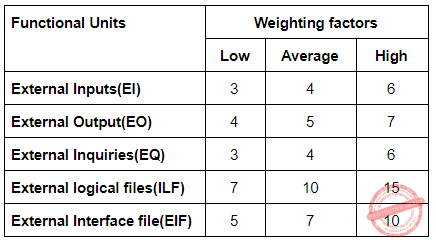Given in question, average weighting factor.
=(External Inputs(EI)*4) + (External Output(EO) * 5) + (External Inquiries(EQ) * 4) + (External logical files(ILF) * 10) + (External Interface file(EIF) * 7)
=(15*4 + 50*5 + 24*4 +12*10 + 8*7
= 582
And, productivity month is 70%
=582*0.7
=407.4
 Question 77
Suppose two jobs, each of which needs 10 minutes of CPU time, start simultaneously. Assume 50% I/O wait time. How long will it take for both to complete, if they run sequentially?
 A 10 B 20 C 30 D 40
Operating-Systems       CPU-Scheduling
Question 77 Explanation:
Given data,
2 jobs need 10 min CPU time,
50% IO waiting time
Step-1: Here, total CPU time is IO operation time and execution time.
Step-2: We can assume, CPU time = (IO operation time*execution time) =2 times
Step-3: CPU running both the process is 10+10 mins.
1 CPU time=20 mins.
Step-4: Need= 2 times *20 min
=40mins.
Note: Actual answer key, they given Option C
 Question 78
If D1, D2…Dn are domains in a relational model, then the relation is a table, which is a subset of
 A D1⊕D2⊕…⊕Dn B D1xD2x…xDn C D1∪D2∪…∪Dn D D1∩D2∩…∩Dn
Database-Management-System       Relational-Calculus
Question 78 Explanation:
In D1,D2,..,Dn are domains in a relational model, then the relation is a table, which s a subset of D1xD2x..xDn
 Question 79
The contents of the flag register after execution of the following program by 8085 microprocessor will be
Program
SUB A
MVI B,(01)H
DCR B
HLT
 A (54)H B (00)H C (01)H D (45)H
Computer-Organization       Microprocessor
Question 79 Explanation:Question 80
The maximum length of an attribute of type text is
 A 127 B 255 C 256 D It is variable
Database-Management-System       Relational-Table
Question 80 Explanation:
Correct answer is D. But actual key they given Option C is correct one.
There are 80 questions to complete.
PHP Code Snippets Powered By : XYZScripts.com